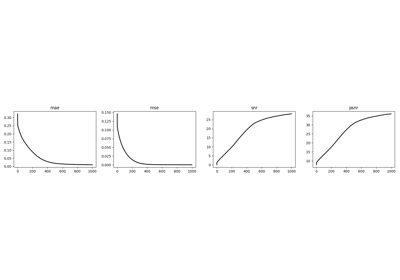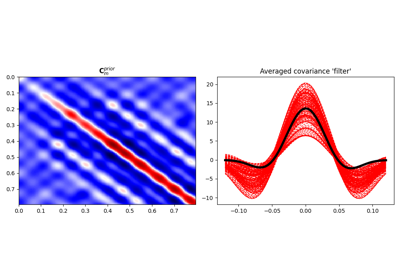# pylops.signalprocessing.FFT#

pylops.signalprocessing.FFT(dims, axis=-1, nfft=None, sampling=1.0, norm='ortho', real=False, ifftshift_before=False, fftshift_after=False, engine='numpy', dtype='complex128', name='F', **kwargs_fftw)[source]#

One dimensional Fast-Fourier Transform.

Apply Fast-Fourier Transform (FFT) along an axis of a multi-dimensional array of size dim.

Using the default NumPy engine, the FFT operator is an overload to either the NumPy numpy.fft.fft (or numpy.fft.rfft for real models) in forward mode, and to numpy.fft.ifft (or numpy.fft.irfft for real models) in adjoint mode, or their CuPy equivalents. When engine='fftw' is chosen, the pyfftw.FFTW class is used instead. Alternatively, when the SciPy engine is chosen, the overloads are of scipy.fft.fft (or scipy.fft.rfft for real models) in forward mode, and to scipy.fft.ifft (or scipy.fft.irfft for real models) in adjoint mode.

When using real=True, the result of the forward is also multiplied by $$\sqrt{2}$$ for all frequency bins except zero and Nyquist, and the input of the adjoint is multiplied by $$1 / \sqrt{2}$$ for the same frequencies.

For a real valued input signal, it is advised to use the flag real=True as it stores the values of the Fourier transform at positive frequencies only as values at negative frequencies are simply their complex conjugates.

Parameters
dimstuple

Number of samples for each dimension

axisint, optional

New in version 2.0.0.

Axis along which FFT is applied

nfftint, optional

Number of samples in Fourier Transform (same as input if nfft=None)

samplingfloat, optional

Sampling step dt.

norm{“ortho”, “none”, “1/n”}, optional

New in version 1.17.0.

• “ortho”: Scales forward and adjoint FFT transforms with $$1/\sqrt{N_F}$$, where $$N_F$$ is the number of samples in the Fourier domain given by nfft.

• “none”: Does not scale the forward or the adjoint FFT transforms.

• “1/n”: Scales both the forward and adjoint FFT transforms by $$1/N_F$$.

Note

For “none” and “1/n”, the operator is not unitary, that is, the adjoint is not the inverse. To invert the operator, simply use Op \ y.

realbool, optional

Model to which fft is applied has real numbers (True) or not (False). Used to enforce that the output of adjoint of a real model is real.

ifftshift_beforebool, optional

New in version 1.17.0.

Apply ifftshift (True) or not (False) to model vector (before FFT). Consider using this option when the model vector’s respective axis is symmetric with respect to the zero value sample. This will shift the zero value sample to coincide with the zero index sample. With such an arrangement, FFT will not introduce a sample-dependent phase-shift when compared to the continuous Fourier Transform. Defaults to not applying ifftshift.

fftshift_afterbool, optional

New in version 1.17.0.

Apply fftshift (True) or not (False) to data vector (after FFT). Consider using this option when you require frequencies to be arranged naturally, from negative to positive. When not applying fftshift after FFT, frequencies are arranged from zero to largest positive, and then from negative Nyquist to the frequency bin before zero.

enginestr, optional

Engine used for fft computation (numpy, fftw, or scipy). Choose numpy when working with cupy arrays.

Note

Since version 1.17.0, accepts “scipy”.

dtypestr, optional

Type of elements in input array. Note that the dtype of the operator is the corresponding complex type even when a real type is provided. In addition, note that neither the NumPy nor the FFTW backends supports returning dtype different than complex128. As such, when using either backend, arrays will be force-casted to types corresponding to the supplied dtype. The SciPy backend supports all precisions natively. Under all backends, when a real dtype is supplied, a real result will be enforced on the result of the rmatvec and the input of the matvec.

namestr, optional

New in version 2.0.0.

Name of operator (to be used by pylops.utils.describe.describe)

**kwargs_fftw

Arbitrary keyword arguments for pyfftw.FTTW

Raises
ValueError
• If dims is provided and axis is bigger than len(dims).

• If norm is not one of “ortho”, “none”, or “1/n”.

NotImplementedError

If engine is neither numpy, fftw, nor scipy.

FFT2D

Two-dimensional FFT

FFTND

N-dimensional FFT

Notes

The FFT operator (using norm="ortho") applies the forward Fourier transform to a signal $$d(t)$$ in forward mode:

$D(f) = \mathscr{F} (d) = \frac{1}{\sqrt{N_F}} \int\limits_{-\infty}^\infty d(t) e^{-j2\pi ft} \,\mathrm{d}t$

Similarly, the inverse Fourier transform is applied to the Fourier spectrum $$D(f)$$ in adjoint mode:

$d(t) = \mathscr{F}^{-1} (D) = \frac{1}{\sqrt{N_F}} \int\limits_{-\infty}^\infty D(f) e^{j2\pi ft} \,\mathrm{d}f$

where $$N_F$$ is the number of samples in the Fourier domain nfft. Both operators are effectively discretized and solved by a fast iterative algorithm known as Fast Fourier Transform. Note that the FFT operator (using norm="ortho") is a special operator in that the adjoint is also the inverse of the forward mode. For other norms, this does not hold (see norm help). However, for any norm, the Fourier transform is Hermitian for real input signals.

Attributes
dimsdtuple

Shape of the array after the forward, but before linearization.

For example, y_reshaped = (Op * x.ravel()).reshape(Op.dimsd).

fnumpy.ndarray

Discrete Fourier Transform sample frequencies

realbool

When True, uses rfft/irfft

rdtypebool

Expected input type to the forward

cdtypebool

Output type of the forward. Complex equivalent to rdtype.

shapetuple

Operator shape

clinearbool

New in version 1.17.0.

Operator is complex-linear. Is false when either real=True or when dtype is not a complex type.

explicitbool

Operator contains a matrix that can be solved explicitly (True) or not (False)

## Examples using pylops.signalprocessing.FFT#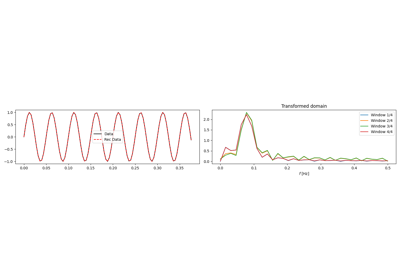1D, 2D and 3D Sliding

1D, 2D and 3D Sliding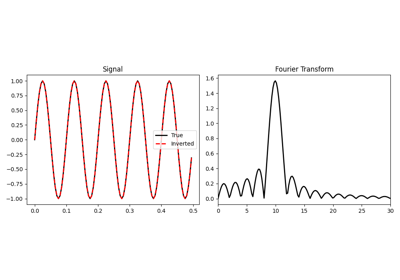Fourier Transform

Fourier Transform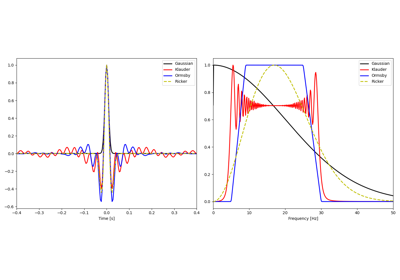Wavelets

Wavelets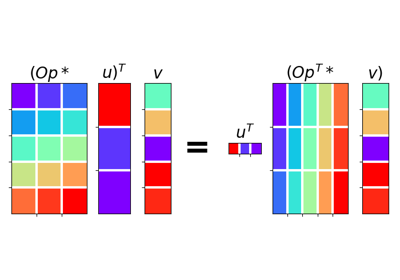02. The Dot-Test

02. The Dot-Test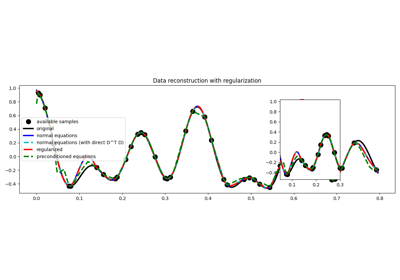03. Solvers

03. Solvers